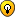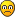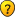## A BIT OF MATHS

Anything goes, but keep it seemly...
Bigtone53 wrote:1. This being a precise scientific matter, obviously the solid cheese went through an ultrascan before the start, as well as an Archimedes-type volume/density analysis.

2. I suspect that it is Swiss cheeses that have the big holes

3. If the cheese was very runny, perhaps some of it gets displaced along with the water when dunked in the bath.

1. That's cleared the original assumption up perfectly

2. Swiss/Dutch - those South American countries always confuse me

3. Excellent point
mikejapan

Posts: 244
Joined: 27 October 2006

wintder wrote:
Bigtone53 wrote:
There are many problems out there which can be simply solved by assuming that there has to be a unique answer or the problem would not be set (shades of UR!). Therefore picking the ideal circumstances to find an answer therefore gives you the answer. My favourite is

A solid spherical cheese has a cylinder of cheese removed from it, leaving a hole through the centre of the cheese from one side to the other with the axis of the hole going through the centre of the former sphere. The hole is 10cm long. What volume of cheese remains?

I am curious about this one. Clearly if there is a unique answer then by using an incredibly thin cylinder the answer would be (4/3)*Pi*(5^3), which is close to 523.6 cm^3

I have no idea on how to answer a larger bore without trying it physically.

wintder, you can calculate this volume using calculus. I've dug out my old highschool maths text book, and worked out the volume of the sphere with the bore removed to be 500*pi/3 cm^3 (no matter what radius it is). So you can assume the radius of the cylinder to be infinitesimal and work out the answer from the whole volume of the sphere, if you have the presumed knowledge that the answer for this problem is independent to the radius of the cylinder.

Bigtone53, you've got to stop posting these types of problems, or you'll have to rename the title of this thread to "A LOT OF MATHS"!udosuk

Posts: 2698
Joined: 17 July 2005

So you can assume the radius of the cylinder to be infinitesimal and work out the answer from the whole volume of the sphere, if you have the presumed knowledge that the answer for this problem is independent to the radius of the cylinder.

My point was that if you assumed that the problem as set had a unique answer (otherwise it would not be set), you could take the most favourable circumstances (the infinitely thin hole) and get the answer directly.

Bigtone53, you've got to stop posting these types of problems

OKBigtone53

Posts: 413
Joined: 19 September 2005

I've made a pic about the calculus required to find the volume of the remaining part, for those who don't want to assume uniqueness:Hopefully it's self-explaining enough.udosuk

Posts: 2698
Joined: 17 July 2005

Nice picture.

to my mind, it is simpler to use integration to work out the volume of the blue bit directly, but I cannot pick any holes in what you say. I could set this out but cannot replicate your symbols for the picture.
Bigtone53

Posts: 413
Joined: 19 September 2005

I wrote:Perhaps next time I will post on how I searched upon all Pythagorean triples within the range 0..49 to find the only 2 possible combinations which satisfy all the constraints. (It isn't that hard, so I don't consider it as brute force.)

Here it is. Remember, from the general configuration, we know that the constraints are like these:
---------------------------------------------------------------------------------
(r2-r1)^2 + a^2 = c^2
(r1+r2)^2 + b^2 = c^2
a, b, c are positive integers
r1, r2 are positive prime integers
c < 50
r1 < r2
a, b are different (which is implied from the fact that r2-r1 < r1+r2)

---------------------------------------------------------------------------------
Firstly, we gather all the Pythagorean triples with the hypotenuse ranging up to 49. There aren't many, just 18:
---------------------------------------------------------------------------------
3,4,5 ; 6,8,10 ; 9,12,15 ; 12,16,20 ; 15,20,25; 18,24,30
21,28,35 ; 24,32,40 ; 27,36,45 ; 5,12,13 ; 10,24,26 ; 15,36,39
8,15,17 ; 16,30,34 ; 7,24,25 ; 20,21,29 ; 12,35,37 ; 9,40,41

---------------------------------------------------------------------------------
From here on in just analyse as below:
Triple click below to see what I wrote:All these triples (x,y,z) satisfy the equation x^2 + y^2 = z^2, so we just need to try each of them to see if they can be (r2-r1,a,c) or (r1+r2,b,c).

Now it's easy to see that both r2-r1 and r1+r2 must be of the same parity (odd or even). Therefore for z to be c we need 2 different x-values or y-values with the same parity. Hence we can eliminate all the triples which only has 1 odd x-value and 1 even y-value (or vice versa) for the z-value.

So we are left with only 8 triples (with 7 different z-values):
z = 10 : x,y = 6,8
z = 20 : x,y = 12,16
z = 25 : x,y = 7,24 or 15,20
z = 26 : x,y = 10,24
z = 30 : x,y = 18,24
z = 34 : x,y = 16,30
z = 40 : x,y = 24,32

For each of these z-values, we take 2 from the x-values and y-values (with the same parity) and assign them as r2-r1 and r1+r2, and work out r1 & r2 to see if they are both prime:

z = 10 : r2-r1=6, r1+r2=8 => r1=1, r2=7
z = 20 : r2-r1=12, r1+r2=16 => r1=2, r2=14
z = 25 : r2-r1=7, r1+r2=15 => r1=4, r2=11
z = 25 : r2-r1=20, r1+r2=24 => r1=2, r2=22
z = 26 : r2-r1=10, r1+r2=24 => r1=7, r2=17
z = 30 : r2-r1=18, r1+r2=24 => r1=3, r2=21
z = 34 : r2-r1=16, r1+r2=30 => r1=7, r2=23
z = 40 : r2-r1=24, r1+r2=32 => r1=4, r2=28

From these 8 triples, the only cases which have both r1 & r2 prime are r1=7, r2=17 and r1=7, r2=23. Hence we know the radius of the small circle must be 7. (Q.E.D.)

(BTW, if we drop the constraints that r1,r2 must be prime, these 8 sets of values are the only possible answers for c<50.)udosuk

Posts: 2698
Joined: 17 July 2005

Bigtone53 wrote:to my mind, it is simpler to use integration to work out the volume of the blue bit directly, but I cannot pick any holes in what you say. I could set this out but cannot replicate your symbols for the picture.

You can find all the symbols I used from the Character Map, available from Accessories -> System Tools. But to make a pretty layout it's not easy. I used Excel to draw the pic.Oops, I've just realised the direct integration of the blue volume is on (R^2-x^2)-(R^2-25) i.e. 25-x^2 over [-5,5] instead of the incorrect (sqrt(R^2-x^2)-sqrt(R^2-25)^2 I thought before. It's very simple indeed. Too long away from calculus.
udosuk

Posts: 2698
Joined: 17 July 2005

If you tilt the diagram 90 degrees could you find the volume of the removed part using just one integral and would that be simpler? (That would be my first approach when faced with such a problem.)
Once upon a time I was a teenager who was active on here 2007-2011
ocean and eleven should have paired up to make a sudoku-solving duo called Ocean's Eleven
999_Springs

Posts: 487
Joined: 27 January 2007
Location: In the toilet, flushing down springs, one by one.

999_Springs wrote:If you tilt the diagram 90 degrees could you find the volume of the removed part using just one integral and would that be simpler? (That would be my first approach when faced with such a problem.)

Obviously you aren't familiar of calculus.The "volume of revolution" requires the integration to be done over the x-axis. If you tilt the diagram 90 degrees you will be calculating the volume of revolution around the y-axis (a "disc" instead of a "cylinder") which will give you an incorrect result.

The direct way to find the volume of the remaining part is to integrate f(x)^2-(R^2-25) over the range of x from -5 to 5.udosuk

Posts: 2698
Joined: 17 July 2005

oops... I actually meant integrating f^2 (x) from -sqrt(x^2-25) to sqrt(x^2-25), but I just realised that what I said would calculate a different volume to the one in question.
I seem to have forgotten everything about calculus since I started doing vectors.
I can't be bothered to get out Character Map to display superscript 2s. Is there a keyboard shortcut for them?
Once upon a time I was a teenager who was active on here 2007-2011
ocean and eleven should have paired up to make a sudoku-solving duo called Ocean's Eleven
999_Springs

Posts: 487
Joined: 27 January 2007
Location: In the toilet, flushing down springs, one by one.

999_Springs wrote:I can't be bothered to get out Character Map to display superscript 2s. Is there a keyboard shortcut for them?

You can find all these keystrokes in the Character Map...udosuk

Posts: 2698
Joined: 17 July 2005

For a wider audience, let me share Victor Bryant's Teaser today in the UK's Sunday Times. I like this one at a number of levels

I keep past Teasers in a properly ordered row of scrapbooks, all equal in size, an odd number of inches thick, including cover, and containing 50 square leaves.
Three bookworms start at the top left hand corner of Page 1 of vol 1 and chew for whole numbers of inches in different directions, The first finishes at the bottom right-hand corner of p100 of vol 2, after an 11 inch journey. the others finish at the bottom corners of p100 of the last volume: their journeys differ by 4 inches.

How many volumes, and how thick is each one?
[/quote]
Bigtone53

Posts: 413
Joined: 19 September 2005

As the chewing is in different directions (then you can't assume a straight line) ....

hmmmtarektarek

Posts: 3351
Joined: 05 January 2006

As the chewing is in different directions (then you can't assume a straight line) ....

hmmm

Tarek, Yoy are quite right and while I did not spot it, the question as originally posed is incomplete. It the worms are chomping around all over the place, then no answer.

But if they are eating in straight linesBigtone53

Posts: 413
Joined: 19 September 2005

That makes it clearerNow ,

My chain of thougts goes as follows

11^2 almost equals (x^2 + x^2)^2 + (2 volume thickness)^2

15^2 almost equals (x^2 + x^2)^2 + (all volume thickness) ^2

15^2 - 11^2 NEARLY EQUALS (all colume thickness)^2 - (2 volume thickness) ^2

I can't figure YET a formula for approximating volume thickness to include covers & Math.ceiling int. but may may be a T&E shouldn't be fartarektarek

Posts: 3351
Joined: 05 January 2006

PreviousNext